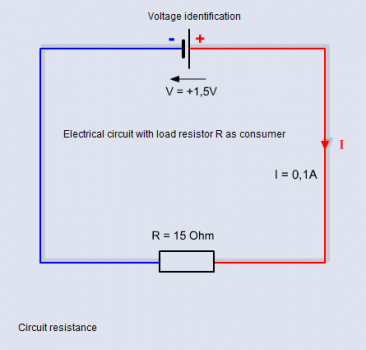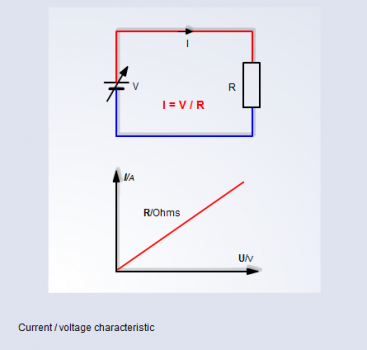# Introduction to Sensor FundamentalsHowever, the current can only flow if the conductor is not interrupted and there are sufficient conducting electrons in the electrical connection between the two poles of the energy (voltage) source.

This impediment to electron flow is the electrical resistance R in Ohms, which is determined by the conducting material and the geometric dimensions of all the components contained in the circuit.

Ohm’s LawIn a circuit the three electrical basic properties of current, voltage and resistance are interdependent. Voltage is responsible for a flowing current.

The amount of current depends on the amount of voltage (proportional) and on the resistance value (inversely proportional) in the entire circuit. The resistance has the effect of limiting the current flow.

Mathematically expressed: I = E / R